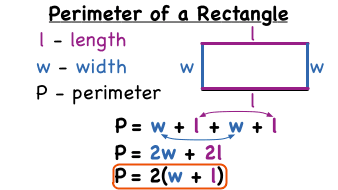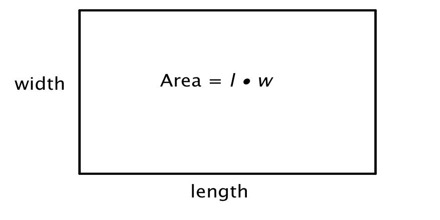Math Puzzles

# It is puzzle time! Can you find the area and perimeter of rectangle?

1.7k views

 1 Introduction 2 Maths puzzles for area and perimeter of rectangle 3 Properties of rectangles 4 Conclusion 5 About Cuemath 6 FAQ's 7 External link

11th November 2020

## Introduction

### Perimeter of rectangle

The perimeter of a rectangle is defined as the sum of all the sides of a rectangle. Like any polygon, the perimeter is the total distance around the outside, which can be found by adding together the length of each side. In the case of a rectangle, opposite sides are equal in length, so the perimeter is twice its length plus twice its width. Or as a formula:

Perimeter    = 2(w+l)

where:

l is the length of the rectangle
w is the width of the rectangleAlso, try:

 Example 1

Find the perimeter of a rectangle whose length and width is 5 cm and 8 cm, respectively.

### Solution:

Given:

Length = 5 cm and Width = 10 cm

We know,

The perimeter of a rectangle = 2(length + width)

Substitute the value of length and width here,

Perimeter, P = 2(5 + 8) cm

P = 2 x 13 cm

Therefore, the perimeter of a rectangle = 26 cm

### Area of rectangle

The area of a rectangle is the product of its two sides, multiplying the length and its width. The unit of area of the rectangle is square units.Example 1

The length and the breadth(width) of a rectangular piece of land are 400 m and 200 m respectively find its area.

### Solution:

The Area of a rectangle (A) = l x w, where l is the length and b are the breadths of the rectangle.

Given, l = 400 m and b = 200 m.

Thus, Area of the rectangle A = 400 m x 200 m = 80,000 square meters.

## Maths puzzles for area and perimeter of rectangle

Math puzzles help children not only gain a deeper insight into mathematical concepts but also strengthen their critical thinking skills. Keeping this in mind, we have designed a fun and engaging math puzzle that will help your child understand math in a fun way.

Using these sheets will help you to solve questions based on area and perimeter:

Math puzzles enable children to visualize concepts, and thus understand math concepts better and faster. Cuemath helps students prepare better by helping them prepare better with over 15000+ workbook problems, 180+ puzzle cards, including several engaging math games that help students understand the ‘why’ behind the ‘what’ of math concepts, thereby enabling them to learn better.

 📥 It is puzzle time! Can you find the area and perimeter of rectangle? Download

## Properties of rectangles

• Rectangles have four sides and four right (90°) angles.
• The lengths of opposite sides are equal.
• The perimeter of a rectangle is the sum of twice the length and twice the width.

2(Length + Width) square units

• The area of a rectangle is the product of the length and the width.

A= a × b

## Conclusion

Did you realize the importance of attempting such puzzles?

By doing this puzzle you have learnt more about measurement, basketball courts, how to measure       radius,  diameter and the area and perimeter of shapes.

Math puzzles help you not only gain a deeper insight into mathematical concepts but also strengthen your critical thinking skills. Keeping this in mind, we have designed a fun and engaging math puzzle that will help you understand math in a fun way.
Hope you had a great learning experience!

Cuemath, a student-friendly mathematics platform, conducts regular Online Live Classes for academics and skill-development and their Mental Math App, on both iOS and Android, is a one-stop solution for kids to develop multiple skills.Understand the Cuemath Fee structure and sign up for a free trial.

## How to find the length and width of a rectangle when given the perimeter and area?

1. Let x= the length and y= the width of the rectangle. The area of the rectangle A=xy.
2. 2x+2y=180 because the perimeter is 180 .
3. Solve for y. 2y=180−2x. y=90−x.
4. Substitute for y in the area equation. A=x(90−x) A=90x−x2.
5. The maximum area is found at x=45. and y=90−x=90−45=45.

## How to Find the Length and Width of a Rectangle When Given the Area?

1. A = L × W A = L × W A=L×W.
2. A = L × W A = L × W A=L×W.
3. P = 2 L + 2 W P = 2L + 2W P=2L+2W.

## Is there a relationship between the area and perimeter of a rectangle?

There is no direct relationship between the perimeter of a rectangle and its area. ... Each of these rectangles has a different perimeter (24 units, 18 units and 42 units respectively). You would need to know a combination of any two of the following: length, width, perimeter, area

Related Articles
Award-winning math curriculum, FREE for a year